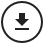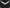# Making work pay in Croatia: An ex-ante evaluation of two in-work benefits using miCROmod

1. Slavko Bezeredi2. Marko Ledić3. Ivica Rubil4. Ivica Urban1. Institute of Public Finance, Croatia
2. Faculty of Economics, Croatia
3. The Institute of Economics, Croatia
Research article
Cite this article as: S. Bezeredi, M. Ledić, I. Rubil, I. Urban; 2019; Making work pay in Croatia: An ex-ante evaluation of two in-work benefits using miCROmod; International Journal of Microsimulation; 12(3); 28-61. doi: 10.34196/ijm.00206
7 figures and 13 tables

## Figures

Figure 1Distributions of discretised hours of work. Source: Authors’ calculations based on the ILCS 2016 data. Notes: The bar height measures the share (in %) of persons working the corresponding number of work hours annually. Sample sizes: 1,444 males from couples, 621 single males, 1,444 females from couples, 423 single females.
Figure 2In-sample prediction performance: observed and predicted distributions of hours of work. Source: Authors’ calculations based on the ILCS 2016 data and the estimates of the labour supply model given in Table 2 (for males and females in couples) and Table 3 (for singles). Notes: The height of each bar labelled “observed” measures the share of males (panel a), females (panel b) or couples (panel c) observed to work the corresponding number of hours annually in 2015. The annual work hours are the hours after discretisation of their actually observed distribution. The height of each bar labelled “predicted” measures the sample average of the probability to work the corresponding number of hours or, in the case of the joint male-female distribution, the corresponding male-female combination of hours. The probabilities are calculated using the parameters of the labour supply model and data for 2015.
Figure 3In-sample prediction performance: observed and predicted densities of total household disposable income. Source: Authors’ calculations based on the ILCS 2016 data and the estimates of the labour supply model given in Table 2 (for males and females in couples) and Table 3 (for singles). Notes: The densities are kernel estimates. The “observed” density refers to the density of total household disposable income actually observed in the 2015 data. The “predicted” density refers to the density of the expected total household disposable income, where the expectation is taken over the alternative-specific incomes, with the choice probabilities as weights. The choice probabilities are calculated using the parameters of the labour supply model and data for 2015.
Figure 4Out-of-sample prediction performance: observed and predicted distributions of hours of work. Source: Authors’ calculations based on the ILCS 2015 data and the estimates of the labour supply model given in Table 2 (for males and females in couples) and Table 3 (for singles). Notes: The height of each bar labelled “observed” measures the share of males (panel a), females (panel b) or couples (panel c) observed to work the corresponding number of hours annually in 2014. The annual work hours are the hours after discretisation of their actually observed distribution. The height of each bar labelled “predicted” measures the sample average of the probability to work the corresponding number of hours or, in the case of joint male-female distribution, the corresponding male-female combination of hours. The probabilities are calculated using the parameters of the labour supply model for 2015 and data for 2014.
Figure 5Out-of-sample prediction performance: observed and predicted densities of total household disposable income. Source: Authors’ calculations based on the ILCS 2015 data and the estimates of the labour supply model given in Table 2 (for males and females in couples) and Table 3 (for singles). Notes: The model is estimated on the 2015 data, and the estimated parameters are used to predict the choice probabilities in the 2014 data. These probabilities are used in the calculation of the expected total disposable income for each household in the 2014 data. The density of this income is labelled “predicted”. The density labelled “observed” is the density of actually observed total household disposable income in the 2014 data.
Figure 6Population aged 20–64 not in employment, education, training, disability or retirement. Source: Authors’ calculations based on Eurostat data on the unemployment rates, the inactivity rates and the structure of inactivity by the main reason.
Figure 7Illustration of the WTC and ETC with two hypothetical couples. a. Spouse A1 will be non-employed all 12 months. Spouse A2 considers obtaining full-time employment for all 12 months b. Spouse B1 will be employed all 12 months, earning 50% of AGW. Spouse B2 considers obtaining full-time employment for all 12 months Notes: Gross income is the sum of the gross employment incomes of both spouses. The participation tax rate is one minus the change in disposable income taking place upon the transition from non-employment to employment, expressed as a share of gross income that would be earned in the case of employment. In panel a., the benefit amount and disposable income at a gross income of zero are identical for the WTC and ETC scenarios; therefore, the markers overlap, i.e., the one showing the WTC scenario is hidden. In Croatia in 2015, the minimum gross wage for full-time employment was approximately 38% of the average gross wage. Therefore, the actors A2 and B2 cannot be legally employed at a gross wage below the minimum wage. Accordingly, we show the results for gross wages beyond 38% of the AGW (represented by full lines and markers on the graphs). However, for the sake of illustration, the points below 38% of the AGW are also shown (represented by dotted lines on the graphs). Source: Authors’ simulations using miCROmod.

Table 1
Table 2
Table 3
Table 4
Table 5
Table 6
Table 7
Table 8
Table 9
Table 10
Table A-1
Table A-2
Table B-1

## Data and code availability

The data is proprietary. The data (referred to as ILCS in the paper) were collected by the Croatian Bureau of Statistics and are available only to Croatian scientific researchers, upon signing a special agreement.

The model is proprietary, with executable also not available.